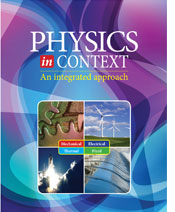# Physics in Context - 2nd Edition

## Chapter 7: MomentumSome links are repeated for use with more than one lesson.

### 7.1  Linear Momentum

Momentum and Its Conservation
http://www.physicsclassroom.com/class/momentum
These are the Physics Classroom lessons on momentum and its conservation, and includes questions for a self-check of your understanding.

Linear Momentum
http://www.sparknotes.com/testprep/books/sat2/physics/chapter9.rhtml
The series of lessons on the SparkNotes site presents the topic of linear momentum, including impulse, conservation and collisions, and center of mass, followed by practice problems.

Impulse
http://www.regentsprep.org/Regents/physics/phys01/impulse/default.htm
Impulse in stopping a car

Collision Simulation
http://www.walter-fendt.de/ph14e/collision.htm
The applet on this page simulates one dimensional elastic or inelastic collisions between carts with adjustable mass and velocity. (Requires Java.)

Momentum Effects on Aerodynamic Forces
http://www.grc.nasa.gov/WWW/K-12/airplane/presar.html
This NASA site discusses momentum effects on aerodynamic forces. It includes a link to a Java simulation that allows you to investigate how an aircraft wing produces lift by changing the values of different factors that affect it.

Momentum and Impulse
http://www.bitesizephysics.com/Lessons/momentum.html
This page at the Bite-Size Physics site discusses and suggests several activities to demonstrate the concepts of impulse and momentum, followed by some practice questions.

How Air Bags Work
http://www.howstuffworks.com/airbag.htm
A discussion from How Stuff Works about air bags in automobiles. The second page deals with momentum in auto accidents.

A simulation of the familiar “Newton's cradle,” with which students can observe the conservation of momentum. (Requires Java.)

The Physics of Football
http://www.howstuffworks.com/physics-of-football4.htm
This page from the How Does the Physics of Football Work? site discusses how tackling and blocking runners rely on three important principles of physics: impulse, conservation of momentum, and rotational motion.

The Physics of Bouncing Balls
http://www.phy.davidson.edu/StuHome/chgreene/BouncingBalls/Final_Project.bouncingBalls.BouncingBalls.html
A more advanced view of two balls bouncing "vertically in line" with each other under the effect of gravity. The user can vary the ratio of the two masses, plotting points for v to x. The Poincare graph appears to be "chaotic" as slight changes in the ratio of masses produce some very interesting patterns on the graph (unclick the "animation" to speed the graph generation).

Explosions and Momentum
http://www.regentsprep.org/Regents/physics/phys01/expmomen/default.htm
Conservation of momentum in explosions

Gun Recoil
http://www.bsharp.org/physics/recoil
This page discusses the conservation of momentum that occurs during a gunshot.

Collision Lab Simulation
This PhET page links to an applet that simulates collisions of air-hockey pucks in 1-dimension or 2-dimensions (click the Advanced tab), as well as links to several learning resources that make use of this applet. Explore the changing energies and momenta with different masses, velocities, and numbers of pucks. (Requires Java.)

### 7.2  Angular Momentum

Angular Momentum
http://hyperphysics.phy-astr.gsu.edu/hbase/amom.html
This page summarizes angular momentum, both for spinning and orbiting masses. It also compares angular and linear momentum formulas.

Conserving Angular Momentum on the Trapeze
http://www.pbs.org/opb/circus/classroom/circus-physics/angular-momentum/
This site includes video that shows how trapeze artists and ice skaters use angular momentum to achieve high speed rotations.

Calculating the Moment of Inertia for Rotating Masses
http://www.dummies.com/how-to/content/how-to-calculate-the-momentum-of-inertia-for-diffe.html
This page presents a concise introduction and presentation of rotational inertia for masses of different shapes, including a reference table of formulas for common shapes.

Angular Momentum and Area
http://www.phy.ntnu.edu.tw/ntnujava/index.php?topic=147
The simulations on this page demonstrate how angular momentum is related to the geometric area “swept out” over time, and so an object moving in a straight line actually demonstrates angular momentum, too. (Requires Java.)

Black Holes
http://phys.org/news/2014-02-fast-black-holes.html
This article and video discusses how black holes must spin faster and faster as they collapse to conserve angular momentum, but that spinning has other consequences.